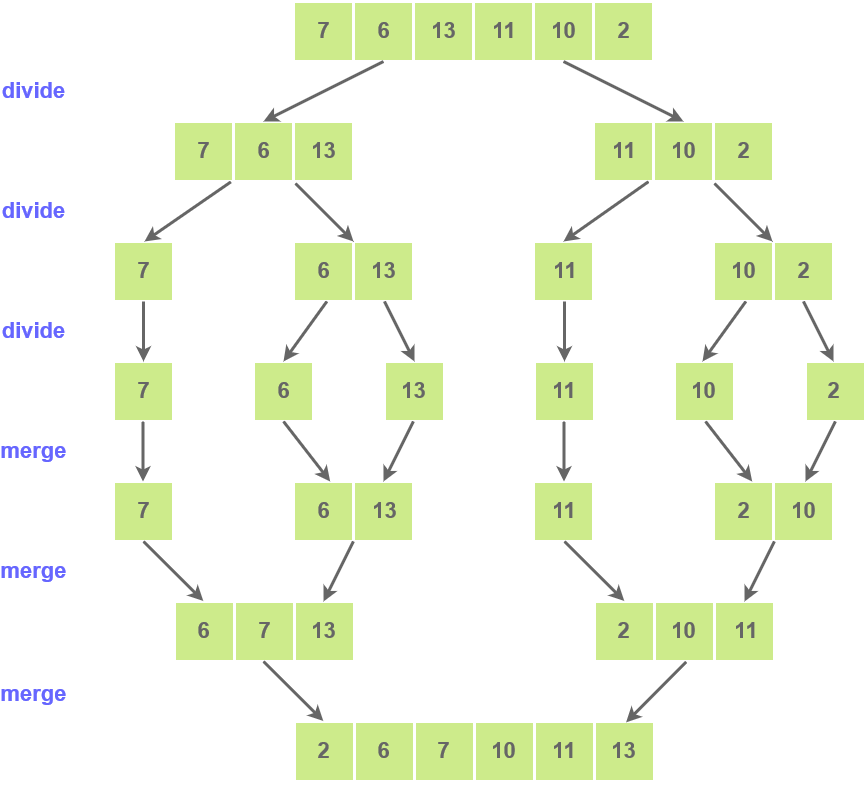# Merge Sort

## Merge Sort

Merge sort is a sorting algorithm that works on the principle of Divide and Conquer. The array or list of elements is repeatedly broken down into several subarrays until each subarray consists of a single element. Then these subarrays are merged in a way such that the result is a sorted list.

Since the merge sort algorithm uses recursion to sort a given set of elements, it consumes less time.

Steps

1. Store the starting index of thearray in `p` and store the last index in `r`.
2. Store the middle index of array in `q` = `(p + r)/2`
3. Break the array into two subarrays, from `p` to `q` and from `q + 1` to `r` index.
4. Divide these 2 subarrays again, just like the main array was divided.
5. Continue dividing into subarrays until subarrays of single elements get created.
6. Now start merging the arrays.Code

``````void MergeSort(int A[], int p, int r)
{
int q;
if(p < r)
{
q = (p + r) / 2;
MergeSort(A, p, q);
MergeSort(A, q+1, r);
merge(A, p, q, r);
}
}

// function to merge the subarrays
void merge(int A[], int p, int q, int r)
{
int B;   //sAme size of A[]
int i, j, k;
k = 0;
i = p;
j = q + 1;
while(i <= q && j <= r)
{
if(A[i] < A[j])
{
B[k] = A[i];
k++;
i++;
}
else
{
B[k++] = A[j++];
}
}

while(i <= q)
{
B[k++] = A[i++];
}

while(j <= r)
{
B[k++] = A[j++];
}

for(i=r; i >= p; i--)
{
A[i] = B[--k];
}
}
``````

Scroll to Top

### Full Stack Integrated Bootcamp Free Trial

• Please enter a number from 7000000000 to 9999999999.